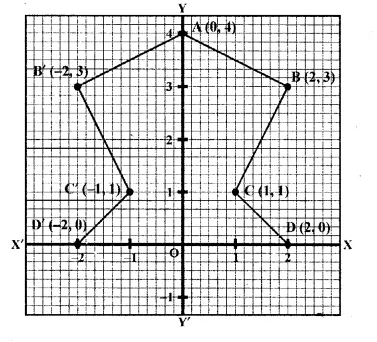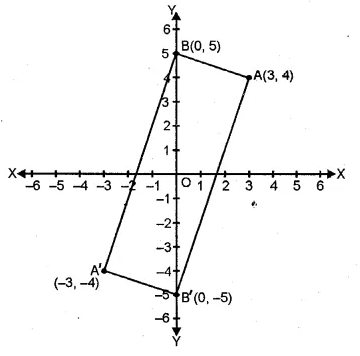## ML Aggarwal Class 10 Solutions for ICSE Maths Chapter 10 Reflection Chapter Test

These Solutions are part of ML Aggarwal Class 10 Solutions for ICSE Maths. Here we have given ML Aggarwal Class 10 Solutions for ICSE Maths Chapter 10 Reflection Chapter Test.

More Exercises

Question 1.
The point P (4, – 7) on reflection in x-axis is mapped onto P’. Then P’ on reflection in the y-axis is mapped onto P”. Find the co-ordinates of P’ and P”. Write down a single transformation that maps P onto P”.
Solution:
P’ is the image of P (4, -7) reflected in x-axis
∴ Co-ordinates of P’ are (4, 7)
Again P” is the image of P’ reflected in y-axis
∴ Co-ordinates of P” are (-4, 7)
∴ Single transformation that maps P and P” is in the origin.

Question 2.
The point P (a, b) is first reflected in the origin and then reflected in the y-axis to P’. If P’ has co-ordinates (3, – 4), evaluate a, b
Solution:
The co-ordinates of image of P(a, b) reflected in origin are (-a, -b).
Again the co-ordinates of P’, image of the above point (-a, -b)
reflected in the y-axis are (a, -b).
But co-ordinates of P’ are (3, -4)
∴a = 3 and -b = -4
b = 4 Hence a = 3, b = 4.

Question 3.
A point P (a, b) becomes ( – 2, c) after reflection in the x-axis, and P becomes (d, 5) after reflection in the origin. Find the values of a, b, c and d.
Solution:
If the image of P (a, b) after reflected in the x-axis be (a, -b) but it Is given (-2, c).
a = -2, c = -b
If P is reflected in the origin, then its co-ordinates will be (-a, -b), but it is given (d, 5)
∴ -b = 5 ⇒ b = -5
d = -a = -(-2) = 2, c = -b = -(-5) = 5
Hence a = -2, b = -5, c = 5, d = 2

Question 4.
A (4, – 1), B (0, 7) and C ( – 2, 5) are the vertices of a triangle. ∆ ABC is reflected in the y-axis and then reflected in the origin. Find the co-ordinates of the final images of the vertices.
Solution:
A (4, -1), B (0, 7) and C (-2, 5) are the vertices of ∆ABC.
After reflecting in y-axis, the co-ordinates of points will be
A’ (-4, -1), B’ (0, 7), C’ (2, 5). Again reflecting in origin,
the co-ordinates of the images of the vertices will be
A” (4, 1), B” (0, -7), C” (-2, -5)

Question 5.
The points A (4, – 11), B (5, 3), C (2, 15), and D (1, 1) are the vertices of a parallelogram. If the parallelogram is reflected in the y-axis and then in the origin, find the co-ordinates of the final images. Check whether it remains a parallelogram. Write down a single transformation that brings the above change.
Solution:
The points A (4, -11), B (5, 3), C (2, 15) and D (1, 1) are the vertices of a parallelogram.
After reflecting in/-axis, the images of these points will be
A’ ( -4, 11), B’ (-5, 3), C (-2, 15) and D’ (-1, 1).
Again reflecting these points in origin, the image of these points will be
A” (4, -11), B” (5, -3), C” (2, -15), D” (0, -1)
Yes, the reflection of a single transformation is in the x-axis.

Question 6.
Use a graph paper for this question (take 2 cm = 1 unit on both x and y axes).
(i) Plot the following points:
A (0, 4), B (2, 3), C (1, 1) and D (2, 0).
(ii) Reflect points B, C, D on 7-axis and write down their coordinates. Name the images as B’, C’, D’ respectively.
(iii) Join points A, B, C, D, D’, C’, B’ and A in order, so as to form a closed figure. Write down the equation of line of symmetry of the figure formed. (2017)
Solution:
(i) On graph A (0, 4), B (2, 3), C (1, 1) and D (2, 0)
(ii) B’ = (-2, 3), C’ = (-1, 1), D’ = (-2, 0)The equation of the line of symmetry is x = 0

Question 7.
The triangle OAB is reflected in the origin O to triangle OA’B’. A’ and B’ have coordinates ( – 3, – 4) and (0, – 5) respectively.
(i) Find the co-ordinates of A and B.
(ii) Draw a diagram to represent the given information.
(iii) What kind of figure is the quadrilateral ABA’B’?
(iv) Find the coordinates of A”, the reflection of A in the origin followed by reflection in the y-axis.
(v) Find the co-ordinates of B”, the reflection of B in the x-axis followed by reflection in the origin.
Solution:
∆ OAB is reflected in the origin O to ∆ OA’B’,
Co-ordinates of A’ = (-3, -4), B’ (0, -5).
.’. Co-ordinates of A will be (3, 4) and of B will be (0, 5).
(ii) The diagram representing the given information has been drawn here.
(iii) The figure in the diagram is a rectangle.
(iv) The co-ordinates of B’, the reflection of B is the x-axis is (0, -5)
and co-ordinates of B”, the reflection in origin of the point (0, -5) will be (0, 5).
(v) The co-ordinates of the points, the reflection of A in the origin are (-3, -4)
and coordinates of A”, the reflected in y-axis of the point (-3, – 4) are (3, -4)Hope given ML Aggarwal Class 10 Solutions for ICSE Maths Chapter 10 Reflection Chapter Test are helpful to complete your math homework.

If you have any doubts, please comment below. Learn Insta try to provide online math tutoring for you.